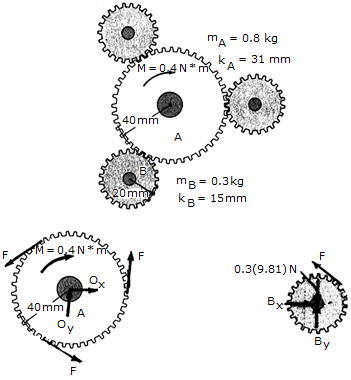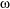# Engineering Mechanics - PKRB: Impulse and Momentum - Discussion

### Discussion :: PKRB: Impulse and Momentum - General Questions (Q.No.6)

6.A constant torque or twist of M = 0.4N • m is applied to the center gear A. If the system starts from rest, determine the angular velocity of each of the three (equal) smaller gears in 3 s. The smaller gears (B) are pinned at their centers, and the mass and centroidal radii of gyration of the gears are given in the figure.

 [A].B = 1975 rad/s [B].B = 5830 rad/s [C].B = 1520 rad/s [D].B = 1022 rad/s

Explanation:

No answer description available for this question.